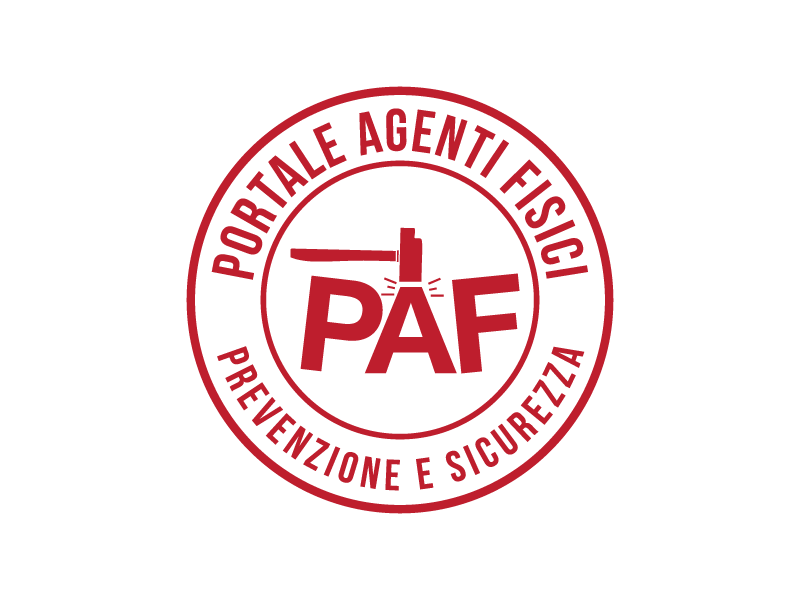# Introduction to the calculation of Protective Equipment for welding as a function of the distance

## "USL Toscana Sudest" Tuscany Agency- Public Health Laboratory of Siena

The following calculation sheet is intended to help for the choice of PPEs for all operators that have to work near a welding station, and that , even if not directly involved in the welding operations, are subject to the risk of exceeding the limits of exposure to optical radiation for the eyes and the skin: this can, e.g. happen in those situations in which it is not possible to adequately shield the welding stations, or when the worker needs to stand near the welding area within a shielded area.

The relevant quantities for such kind of exposure are:

• the UV(S) effective irradiance; target organs: anterior chamber of the eye and skin
• the irradiance in the UVA region; target organ: eye
• the irradiance weighted by B curve (blue light damage); target organ: retina.

Given the typical emission spectrum of arc welding (usually centred in the visible and UV bands), it is assumed that the retinal thermal damage is negligible if the photochemical damage (due to blue light) is prevented; therefore, the relative quantity LR is not taken into account for the purposes of calculation.

In order to assess the blue light hazard, we consider the total irradiance to which the eye is exposed, in the hypothesis of a point source; this is true if the angle subtended by the source is below 11mrad. Considering an electrical welding arc having a length of few millimetres and a thickness of fractions of a millimetre, this hypothesis is verified even at a distance of 1 meter; all the more reason the hypothesis will be verified for operators that are not directly involved in the welding process and that are likely to stay at a distance above 1 meter from the welding station.

In the first part of the calculator the UV(S), UVA and blue light data - as they are obtained in the database - must be inserted: in this case the welding typology and the operational characteristics that are the subject of the calculation must be selected in the database.

As an alternative, the inserted values can be derived from direct measurement by using a broadband radiometer or spectrometer and by applying the related spectral weighting functions.

The distance at which the above-mentioned irradiance values have been acquired and the distance for which the calculation has to be performed (distance at which the operators to be protected stand from the welding source) are also required.

In the two following boxes one can choose the shade numbers of PPEs for welding (EN 169) and ultraviolets (EN 170) in the respective drop-down menus. The first have to be used when one wants to choose a PPE for a worker involved, in any possible way, in the welding process, the second menu (EN 170) allows to choose the protective goggles for an operator that is not in charge of welding.

Each box displays, for each chosen shade number, the corresponding transmission value used in the calculation. t-313, t-365, tV, e tA. These values can also be found in the system tables displayed at the bottom of the calculator. These values (derived by the standards) are used to estimate the transmission of eye PPEs in the UV (UVC+UVB+UVA), UVA and visible (for blue light) regions and the resulting attenuations of the three considered irradiances (UV(S), UVA, Blue).

By proceeding in the "non attenuated" column, for each kind of irradiance the following values are given:

• the exposure value for the operator calculated at the chosen distance: considering the source as a point source, this value is estimated by using the 1/d2 law: when the distance doubles, the values of the quantity becomes ¼ of the preceding one;
• the percent of the exposure (calculated in the preceding point) compared to the limit value. In the case of UV(S) and UVA, as the limit is given in terms of dose over 8 hours, the limit value in terms of irradiance has been determined by assuming a 8-hours continuous exposure.
• the maximum time of exposure that allows not to exceed the Exposure Limit Value (ELV) for exposures up to 8 hours: if the result gives a maximum time of exposure above 8 hours the provided result will be:  > 8 hours

In the subsequent columns the same quantities are calculated, with the irradiance values adequately attenuated by using the attenuation factors associated with the shade number chosen for the PPE and set by the specific construction standard (EN 169 or EN 170). On the basis of these attenuation values, the calculation program provides the assessment of the percentage of eye exposure compared to the ELV and the maximum time of exposure when wearing the PPE.

It must be observed that the exposure times to be considered when assessing if the limit values are exceeded refer, for the blue light risk, to the actual time spent by the operator staring at the source, whereas, for UV(S) and UVA damages, they refer to the time during which the source is within the field of vision, even if there is no direct staring by the operator.

Therefore, the maximum values concerning the times of exposure to blue light provided by the present calculator must be intended for staring directly at the source: usually this is part of the visual task only for those directly in charge of welding.

In this respect it should be noted that both ICNIRP and D.lgvo 81/2008 consider as safe under non-staring conditions a source for which the exposure limit value in the visible range is exceeded for a time of exposure above 100 seconds.

The program always notifies the need to protect the skin (photoexposed areas) if the limit value for the exposure to the ultraviolet UV(S) is exceeded.